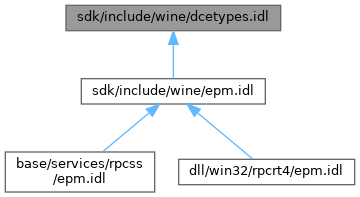ReactOS 0.4.15-dev-6657-ged9973f
dcetypes.idl File Reference
This graph shows which files directly or indirectly include this file:Go to the source code of this file.

## Classes

struct  twr_t

struct  ndr_context_handle

struct  ndr_format_t

struct  rpc_if_id_t

struct  rpc_if_id_vector_t

struct  rpc_stats_vector_t

## Typedefs

typedef unsigned small unsigned8

typedef unsigned short unsigned16

typedef unsigned long unsigned32

typedef small signed8

typedef short signed16

typedef long signed32

typedef unsigned32 boolean32

typedef unsigned long u_int32

typedef unsigned short u_int16

typedef unsigned small u_int8

typedef GUIDuuid_p_t

typedef struct twr_t twr_t

typedef twr_ttwr_p_t

typedef struct ndr_context_handle ndr_context_handle

typedef struct ndr_format_tndr_format_p_t

typedef rpc_if_id_trpc_if_id_p_t

typedef rpc_if_id_vector_trpc_if_id_vector_p_t

typedef struct rpc_stats_vector_trpc_stats_vector_p_t

## Variables

const long ndr_c_int_big_endian = 0

const long ndr_c_int_little_endian = 1

const long ndr_c_float_ieee = 0

const long ndr_c_float_vax = 1

const long ndr_c_float_cray = 2

const long ndr_c_float_ibm = 3

const long ndr_c_char_ascii = 0

const long ndr_c_char_ebcdic = 1

## ◆ boolean32

 typedef unsigned32 boolean32

Definition at line 30 of file dcetypes.idl.

## ◆ ndr_format_p_t

 typedef struct ndr_format_t * ndr_format_p_t

## ◆ rpc_if_id_p_t

 typedef rpc_if_id_t* rpc_if_id_p_t

Definition at line 74 of file dcetypes.idl.

## ◆ rpc_if_id_vector_p_t

 typedef rpc_if_id_vector_t* rpc_if_id_vector_p_t

Definition at line 82 of file dcetypes.idl.

## ◆ rpc_stats_vector_p_t

 typedef struct rpc_stats_vector_t * rpc_stats_vector_p_t

## ◆ signed16

 typedef short signed16

Definition at line 28 of file dcetypes.idl.

## ◆ signed32

 typedef long signed32

Definition at line 29 of file dcetypes.idl.

## ◆ signed8

 typedef small signed8

Definition at line 27 of file dcetypes.idl.

## ◆ twr_p_t

 typedef twr_t* twr_p_t

Definition at line 43 of file dcetypes.idl.

## ◆ twr_t

 typedef struct twr_t twr_t

## ◆ u_int16

 typedef unsigned short u_int16

Definition at line 33 of file dcetypes.idl.

## ◆ u_int32

 typedef unsigned long u_int32

Definition at line 32 of file dcetypes.idl.

## ◆ u_int8

 typedef unsigned small u_int8

Definition at line 34 of file dcetypes.idl.

## ◆ unsigned16

 typedef unsigned short unsigned16

Definition at line 25 of file dcetypes.idl.

## ◆ unsigned32

 typedef unsigned long unsigned32

Definition at line 26 of file dcetypes.idl.

## ◆ unsigned8

 typedef unsigned small unsigned8

Definition at line 24 of file dcetypes.idl.

## ◆ uuid_p_t

 typedef GUID* uuid_p_t

Definition at line 36 of file dcetypes.idl.

## ◆ ndr_c_char_ascii

 const long ndr_c_char_ascii = 0

Definition at line 57 of file dcetypes.idl.

## ◆ ndr_c_char_ebcdic

 const long ndr_c_char_ebcdic = 1

Definition at line 58 of file dcetypes.idl.

## ◆ ndr_c_float_cray

 const long ndr_c_float_cray = 2

Definition at line 55 of file dcetypes.idl.

## ◆ ndr_c_float_ibm

 const long ndr_c_float_ibm = 3

Definition at line 56 of file dcetypes.idl.

## ◆ ndr_c_float_ieee

 const long ndr_c_float_ieee = 0

Definition at line 53 of file dcetypes.idl.

## ◆ ndr_c_float_vax

 const long ndr_c_float_vax = 1

Definition at line 54 of file dcetypes.idl.

## ◆ ndr_c_int_big_endian

 const long ndr_c_int_big_endian = 0

Definition at line 51 of file dcetypes.idl.

## ◆ ndr_c_int_little_endian

 const long ndr_c_int_little_endian = 1

Definition at line 52 of file dcetypes.idl.